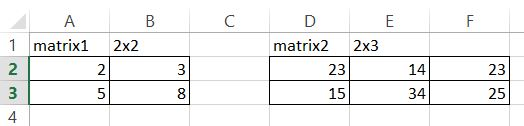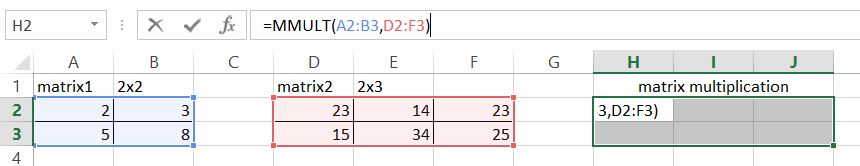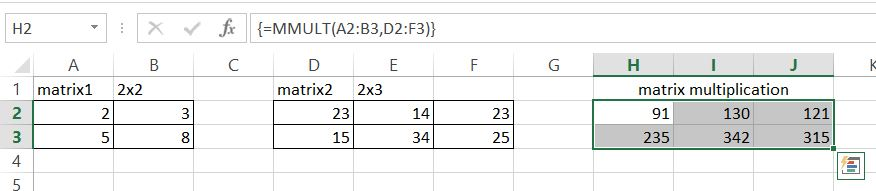#### Matrix multiplication in Excel

In this Excel tutorial you teach yourself about matrix multiplication in Excel. You see how to use MMULT Excel function to multiply matrixes.

A matrix is a rectangular array of numbers, symbols, and expressions arranged in rows and columns. They are used to save large data sets that are in a relationship to each other. Matrices play a particularly important role in computer science and statistics, where huge data sets are often present. For our case matrixes have to contain just numbers.

## Rules of calculations

There are a few rules to follow when you multiply matrices.

1. To be able to multiply the first matrix (let’s call it matrix A) needs to have the same number of rows as the number of columns of the second matrix (let’s call it matrix B).
2. Once we have the appropriate 2 matrices, what can we expect the resulting matrix (matrix C). It will have the size of the number of rows in matrix A and the number of columns in matrix B.

Here are my sample matrixes. Matrix A is 2×2 and Matrix is 2×3. The calculation is possible because the number of rows of matrix A is the same as the number of columns of matrix B. The numbers of columns of matrix A and rows of matrix B do not matter at all.## MMULT function

Then you need to use MMULT Excel function to multiply given arrays. We will use MMULT Excel function for matrix multiplication.

### The syntax of MMULT function

=MMULT (array1, array2)

MMULT function takes arrays as arguments so it is obvious to use.

#### Arguments

array1 – The first array to multiply

array2 – The second array to multiply.

For example, if you have two arrays, A1:B2 and C1:D2, you can multiply these arrays with the following formula:

=MMULT(A1:B2,C1:D2)

The result of the formula will be placed in the top-left cell of the resulting array, and the rest of the cells in the array will be automatically filled in.

## Matrix multiplication

To use it, highlight the whole matrix and type formula in first cell.

MMULT formula in my example is =MMULT(A2:B3,D2:F3)This is an array formula so press CTRL + SHIFT + ENTER keyboard shortcut.This is how multiplied matrix looks like.

## Matrix multiplication using VBA code

You can perform matrix multiplication in Microsoft Excel using Visual Basic for Applications (VBA) code. Here’s an example of how you can write a function to multiply two matrices in VBA:

`Function MatrixMultiplication(matrix1 As Variant, matrix2 As Variant) As Variant `

` ' Check if the number of columns in matrix1 matches the number of rows in matrix2 `

` If (UBound(matrix1, 2) <> UBound(matrix2, 1)) Then `

`  MatrixMultiplication = "Error: Matrices cannot be multiplied" `

`  Exit Function `

` End If `

` Dim result As Variant `

` ReDim result(0 To UBound(matrix1, 1), 0 To UBound(matrix2, 2)) `

` Dim i As Integer `

` Dim j As Integer `

` Dim k As Integer `

` For i = 0 To UBound(matrix1, 1) `

`  For j = 0 To UBound(matrix2, 2) `

`   result(i, j) = 0 `

`   For k = 0 To UBound(matrix1, 2) `

`    result(i, j) = result(i, j) + matrix1(i, k) * matrix2(k, j) `

`   Next k `

`  Next j `

` Next i `

` MatrixMultiplication = result `

`End Function`

You can use this function in your Excel worksheet by calling the MatrixMultiplication function and passing the two matrices as arguments. For example:

`=MatrixMultiplication(A1:B2,C1:D2)`
```Further reading: